# Topic: Chemical Kinetics (Test 1)

Topic: Chemical Kinetics
Q.1
Study of rate of chemical reaction is known as
A. Chemical change
B. Chemical thermodynamics
C. Chemical equilibrium
D. Chemical kinetics
Explaination / Solution:

Chemical Kinetics deals with rate of a Chemical reactions.

Workspace
Report
Q.2
Which among the following is an instantaneous reaction?
A. Hydrolysis of starch
B. Burning of a log of wood
C. Rusting of iron
D. Precipitation of silver chloride
Explaination / Solution:

Precipitation of AgCl is an example of instantaneous reaction.

Workspace
Report
Q.3
The rate of reaction can be measured as
A. Rate of appearance of reactant
B. Both (b) and (c).
C. Rate of disappearance of reactant
D. Rate of appearance of the product
Explaination / Solution:

Rate of reaction can be measured in terms of Rate of disappearance of reactant or rate of apperance of product.

Workspace
Report
Q.4
Rate of reaction for the combustion of propane is equal to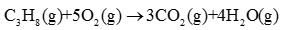A.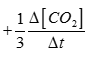B.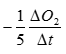C.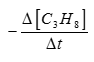D. All of these
Explaination / Solution: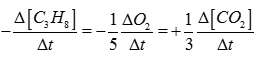Workspace
Report
Q.5
What is the order of a reaction which has a rate expression ; Rate =
A. 12
D. 32
Explaination / Solution:

Total order of reaction = order wrt to A + order wrt to B.

Workspace
Report
Q.6
Molecularity of a reaction
A. Is always a whole number
B. Can have a fractional values
C. Cannot be less than 2
D. Can be zero
Explaination / Solution:

Molecularity means minimum no. of atoms molecules or ions which undergo collision for conversion of reactant to Product. So it is a whole no. Cannot be fractional or negative.

Workspace
Report
Q.7
For which of the following reaction the units of rate constant and rate of the reaction are same?
A. Zero order reaction
B. Third order reaction
C. First order reaction
D. Second order reaction
Explaination / Solution:

Rate=k[Reactant]0
Workspace
Report
Q.8
Which of the following statement is true for order of a reaction?
A. It can have fractional value
B. It cannot be determined experimentally
C. It can be written from the balanced chemical equation
D. It depends on the stiochiometric coefficients of reactants as well as products
Explaination / Solution:

Order of a reaction is experimentally determined quantity so it can be negative fractional or zero.

Workspace
Report
Q.9
For a reaction; pA +qB products, the rate law expression is
A. (p+q) > (l+m)
B. (p+q) may or may not be equal to (l+m)
C. (p+q) = (l+m)
D. (p+q) ≠ (l+m)
Explaination / Solution:

Order of a reaction is experimentally determined quantity. It may or may not be equal to stoichiometric coefficients.

Workspace
Report
Q.10
For the first order reaction, half life is equal to
A. [Ro]K
B.  2.303[Ro]K
C.  0.693K
D. [Ro]2K